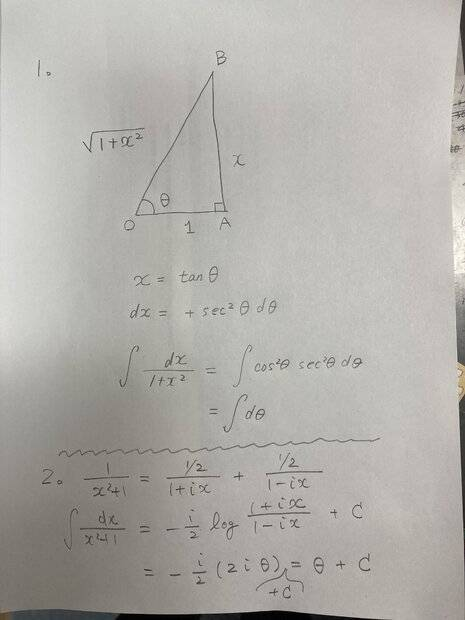# Integral of 1 / (x^2 + 2) dx ?

I think the issue is for the OP to do the integration and not just look it up as a 'standard' integral. My point is if you do the integration on both, they are the same difficulty are they...?f
Is there some simple easy trick I am missing that makes this much easier than ? You can tell me privately if you wish.
Please take a look at attached calculation.Last edited by a moderator:
•bob012345
Please take a look at attached calculation.
Thanks. It does seem simpler with the ##1## in the denominator.

Last edited:
@bob012345, multiply the identity ##\dfrac{1}{(x+3i)(x-3i)} = \dfrac{A}{x + 3i} + \dfrac{B}{x-3i}## by the factor ##(x-3i)##, and then set ##x = 3i## (you can do this because the identity holds for all ##x##). And do a similar thing to find ##A##.
But for x=3i makes some of the denominators of the identity zero...

But for x=3i makes some of the denominators of the identity zero...
If you multiply both sides by one of the factors, say ##x-3i##, solve for ##A## and then set ##x=3i## it works. Cancel those terms before you make the substitution.

But for x=3i makes some of the denominators of the identity zero...
That's the idea, so you can find A, then do x=-3i and find B.

That's the idea, so you can find A, then do x=-3i and find B.
I think it is being suggested this is like the proof ##1=2##.

If you multiply both sides by one of the factors, say ##x-3i##, solve for ##A## and then set ##x=3i## it works. Cancel those terms before you make the substitution.
Emmm, by multiplying both sides by (x-3i) and then making x=3i, you will not fall into any error, it is valid to do so.

It should not have been left to the reader to do first the multiplication of the identity by x-3i, it should have been explicitly mentioned by @ergospherical :D.

But for x=3i makes some of the denominators of the identity zero...
You can think of it like this:
\begin{align*}
\lim_{x \to 3i} (x-3i)\frac{1}{(x-3i)(x+3i)} &= \lim_{x \to 3i} (x-3i)\left[\frac{A}{x-3i}+\frac{B}{x+3i}\right] \\
\lim_{x \to 3i} \frac{1}{x+3i} &= \lim_{x \to 3i} \left[A+B\frac{x-3i}{x+3i}\right] \\
\end{align*} After canceling the factors of ##x-3i##, the functions are continuous at ##x=3i##, so you can evaluate the limit by simply plugging in the value.

•SammyS
Please take a look at attached calculation.
This is exactly how I would do it.

I think it is being suggested this is like the proof ##1=2##.
No, not at all. I think you might be referring to the post by ergospherical.
ergospherical said:
multiply the identity ##\dfrac{1}{(x+3i)(x-3i)} = \dfrac{A}{x + 3i} + \dfrac{B}{x-3i}## by the factor ##(x-3i)##, and then set ##x = 3i## (you can do this because the identity holds for all ##x##). And do a similar thing to find ##A##.
An important point is that the equation above is an identity: one that is is true for all values of the variable x, excepting only ##x = \pm 3i##. For any values of x other than these two, we can multiply both sides of the equation by ##(x + 3i)(x - 3i)## to get this equation:
##1 = A(x - 3i) + B(x + 3i)##
The new equation is still an identity: it must be true for any value of x. The way I chose to go is to solve for A and B like so:
##1 = (A + B)x - 3i(A - B)
For this to be true for all values of x, A + B must be 0 (there is no x term on the left side), and -3i(A - B) = 1.
Solve these two equations for A and B, and Bob's your uncle.

Last edited:
•benorin, bob012345 and Tapias5000
It should not have been left to the reader to do first the multiplication of the identity by x-3i, it should have been explicitly mentioned by @ergospherical :D.
hehe, I use Quora more so my answers are usually complete aum, I'm not used to this forum at all hehe.Thanks. It does seem simpler with the ##1## in the denominator.
Then
$$\int \frac{dx}{x^2+2}= \frac{1}{\sqrt{2}}\int\frac{d\frac{x}{\sqrt{2}}}{(\frac{x}{\sqrt{2}})^2+1}=...$$

Last edited:
•benorin and Delta2
Then
$$\int \frac{dx}{x^2+2}= \frac{1}{\sqrt{2}}\int\frac{d\frac{x}{\sqrt{2}}}{(\frac{x}{\sqrt{2}})^2+1}=...$$
I just used a basic trig with ##tan(\theta)= \large\frac{x}{a}## and it worked fine.

I just used a basic trig with ##tan(\theta)= \large\frac{x}{a}## and it worked fine.
The image in post #36 shows why this substitution works. For ##x^2 + 2##, the length of the leg adjacent to the angle is ##\sqrt 2## rather than 1.

The image in post #36 shows why this substitution works. For ##x^2 + 2##, the length of the leg adjacent to the angle is ##\sqrt 2## rather than 1.
Of course and the point I made was the method I used works for any ##a##. That's why I used ##a## and not ##1##. I found it easier than manipulating the integral first to make it a ##1## or using complex numbers.

Last edited:
Now try it with a hyperbolic trig substitution.

Now try it with a hyperbolic trig substitution.
I tried it and it was not easier by any means.

I think that the most straightforward way is to factorise ##x^2+2## into ##2\left(1+x^2/2\right)=2\left[1+(x/\sqrt 2)^2\right]##.
When you see an integral close to one that you would usually find in an integration table, ##(1+x^2)^{-1}## for instance, try adding a ##0## or factorising something.

•benorin
You guys have beaten this integral in a few ways, OP did you follow any of them? The easy way involves ##1+\left(\tfrac{x}{\sqrt{2}}\right) ^2## and a trig substitution. You probably haven’t learned series methods yet but that would of course be much harder.

I think you can safely lock this thread.